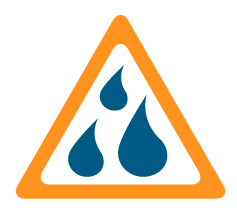# Saturation ConditionsCalculated water vapour pressure higher than saturation vapour pressure.

Increase sample temperature or reduce input humidity.

Calculated water vapour pressure higher than total pressure.

Increase sample pressure or reduce input humidity.

Calculated water vapour or saturation vapour pressure is negative.

Input parameters out of range.

Water Vapour Pressure 0Pa
Saturation Vapour Pressure 0Pa
Dew Point 0°C
Frost point 0°C
Relative Humidity 0
Moisture (Volume) 0ppmV
Moisture (Weight) 0ppmW
Absolute Humidity 0kg/m3
Mixing Ratio 0g/kg
Wet Bulb 0°C
Enthalpy 0kJ/kg
Water Activity 0
Michell Humidity Calculator Settings
Displayed results
Result units
Other options
Psychrometer Constant[?] ×10-4 Blank for Assmann coef.

Help[ Close ]
Known Parameter The humidity parameter that is known. e.g. Dew Point
Known Value The value of the known parameter.
Known Units The unit of measurement of the known parameter. e.g °C
Sample Pressure The pressure at the point where the humidity measurement is made. Defaults to atmospheric pressure.
Pressure Units The unit of measurement of the sample pressure. e.g. Pascals
Pressure Compensation If conversion to another pressure is required, enter that pressure in this box. The measurement units will be the same used for the Sample Pressure.
Gas Type Choose from a list of preset molecular weights. Used only for ppmW & mixing ratio calculations. A user-defined molecular weight can be entered by selecting 'User'.
Mol. Weight Molecular weight of gas being measured, used only for ppmW/Mixing Ratio calculations.
Sample Temperature The temperature at the point where the humidity measurement is made.
Temperature Units The temperature units to use for all temperature parameters including sample temperature, dew-point, frost-point and wet bulb. e.g. °C
WMO Standard for %RH Saturation vapour pressure is calculated over water even for temperatures below freezing as per WMO standard.
Rounding Weight Total number of digits to show when rounding. Default 5.
Psychrometer Coefficient Psychrometer coefficient for wet bulb calculations. Default 6.62 × 10-4